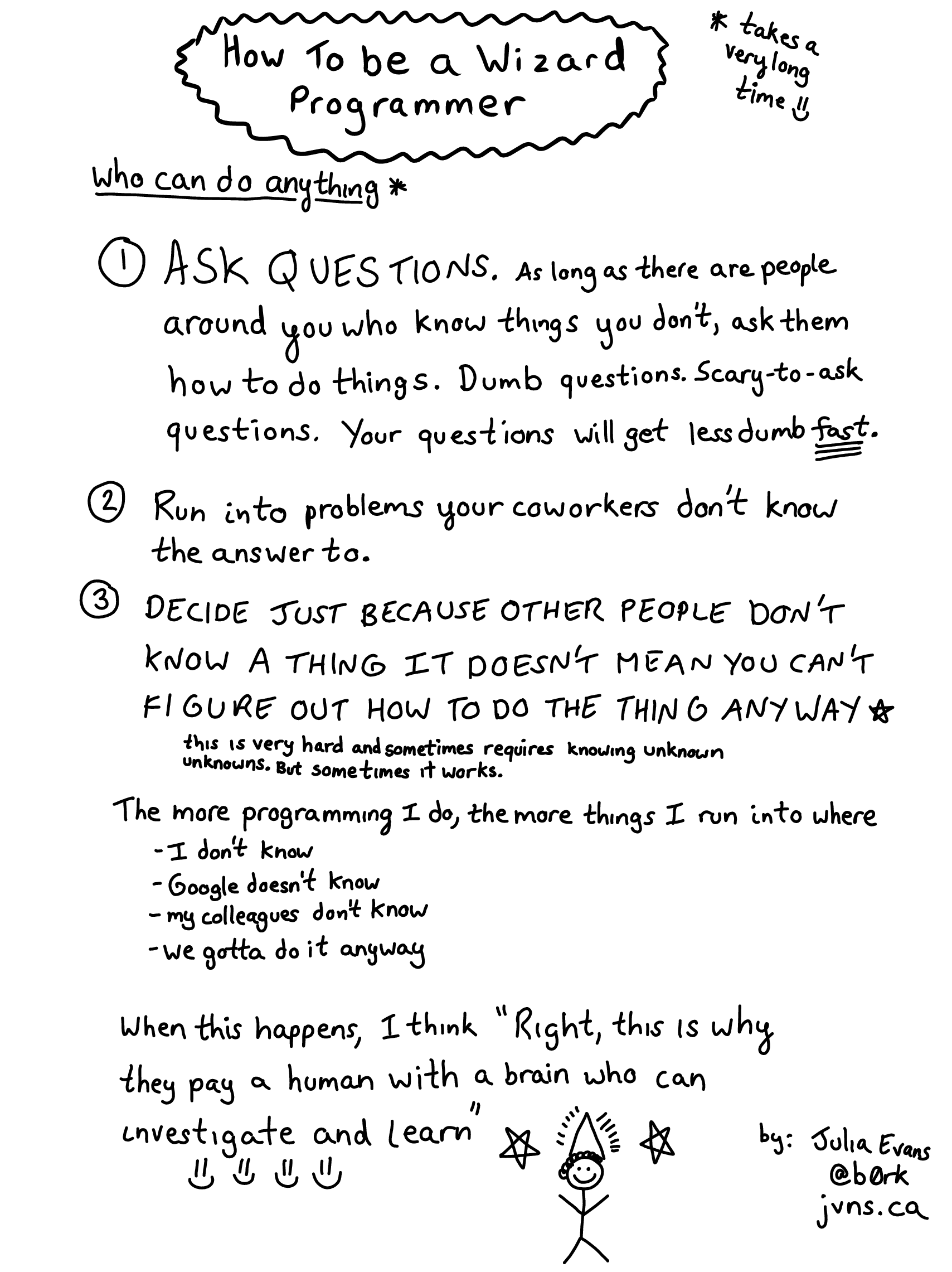# ARTS - Week 1

ARTS 是耗叔发起的一项活动，每周完成以下内容：

Algorithm - 至少做一个 LeetCode 上的算法题
Review - 阅读并点评至少一篇英文技术文章
Tip - 学习至少一个技术技巧
Share - 至少分享一篇有观点和思考的技术文章

## Algorithm

### LeetCode Problems 1 - Two Sum

Given an array of integers, return indices of the two numbers such that they add up to a specific target.

You may assume that each input would have exactly one solution, and you may not use the same element twice.

Example:

Given nums = [2, 7, 11, 15], target = 9,
Because nums + nums = 2 + 7 = 9,
return [0, 1].


#### Solution

##### 第一种方法：暴力循环

# Ruby

def two_sum(nums, target)
for i in 0...nums.size
for j in (i + 1)...nums.size
return [i, j] if nums[i] + nums[j] == target
end
end

raise ArgumentError, 'No two sum solution'
end


• Time complexity: O(n^(2));
• Space complexity: O(1);

Runtime: 5088 ms.
Your runtime beats 3.17 % of ruby submissions.

##### 第二种方法：使用哈希表

#Ruby

def two_sum(nums, target)
hash = {}
nums.each_with_index do |num, index|
return [hash[target - num], index] if hash.has_key?(target - num)
hash[num] = index
end

raise ArgumentError, 'No two sum solution'
end


• Time complexity: O(n);
• Space complexity: O(n). 最坏的情况为数组中的所有元素都需要添加到新的哈希表中，占据新的内存空间;

Runtime: 48 ms.
Your runtime beats 89.40 % of ruby submissions.

### LeetCode Problems 20 - Valid Parentheses

Given a string containing just the characters '(', ')', '{', '}', '[' and ']', determine if the input string is valid.

An input string is valid if:

1. Open brackets must be closed by the same type of brackets.
2. Open brackets must be closed in the correct order.

Note that an empty string is also considered valid.

Example:

Input: "{[]}"
Output: true
Input: "([)]"
Output: false


#### Solution

# Ruby

def is_valid(s)
arr = []
match_hash = {')': '(', ']': '[', '}': '{'}

str_arr = s.split('')
str_arr.each do |char|
if !match_hash.has_key?(char.to_sym)
arr.push(char)
elsif match_hash[char.to_sym] != arr.pop
return false
end
end

return arr.empty?
end


• Time complexity: O(n);
• Space complexity: O(n);

Runtime: 48 ms.
Your runtime beats 69.83 % of ruby submissions.

## Review

For Static Sites, There’s No Excuse Not to Use a CDN

Application-Layer DDoS Attack Protection with HAProxy
Mozilla 基金会的这篇文章简要介绍了浏览器发起 request，并由服务器返回 response 的过程。并就域名解析过程中 DNS 服务器的递归查询做了图文并茂的说明。引出在域名解析过程中可能存在的风险。

• 你的设备使用的域名解析器是网络提供商提供的，是不值得信任的。它会记录你的请求，以及参与伪造从 DNS 返回的信息；
• DNS 服务器和域名解析过程中的路由，都能接触到谁请求了哪个域名的信息。而这些信息是很有价值的，很有可能被其他人拿来获利；
• 利用 DNS 服务器和域名解析过程中的路由，黑客会把用户导向钓鱼网站。

• 使用 TTR 来避免不可信任的解析器；
• 使用 DNS over HTTPS 将 DNS 包加密，使域名解析过程中的路由器无法查看请求的内容；
• 发给每个 DNS 服务器的内容只包含与该服务器相关的部分，而不是像之前一样把所有请求信息都发给所有涉及到的 DNS 服务器。这意味着每个服务器能查看到的信息是不完整的。

## Tip

class Node
attr_accessor :value, :next

def initialize(value, next_node)
@value = value
@next = next_node
end
end

else
@tail.next = Node.new(value, nil)
@tail = @tail.next
end
end

def display
arr = []
while(curr != nil)
arr.push(curr.value)
curr = curr.next
end
p arr
end
end


## Share# ARTS